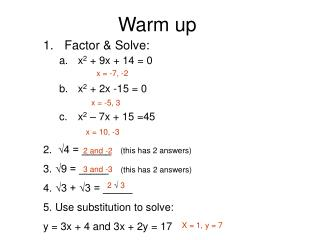DownloadDownload PresentationWarm up

# Warm up

Download Presentation## Warm up

- - - - - - - - - - - - - - - - - - - - - - - - - - - E N D - - - - - - - - - - - - - - - - - - - - - - - - - - -
##### Presentation Transcript

1. Warm up x = -7, -2 x = -5, 3 x = 10, -3 Factor & Solve: x2 + 9x + 14 = 0 x2 + 2x -15 = 0 x2 – 7x + 15 =45 • 2. √4 = _____ (this has 2 answers) • √9 = _____ (this has 2 answers) • √3 + √3 = _____ • Use substitution to solve: • y = 3x + 4 and 3x + 2y = 17 2 and -2 3 and -3 2 √ 3 X = 1, y = 7

2. 3.2 Properties of Quadrilaterals Any four sided polygon is a quadrilateral. We’ll study special quadrilaterals in this section: Trapezoid Trapezium Parallelogram Rhombus Rectangle Square Kite

3. Properties of a TRAPEZIUM:A quadrilateral with NO parallel sides. • 2 pair of consecutive congruent sides • Opposite sides are NOT congruent • Diagonals are perpendicular • Notice only ONE diagonal is bisected A special Trapezium is a KITE

4. Properties of a trapezoid • A trapezoid has one and only one pair of parallel sides.

5. supplementary Properties of parallelograms • Opposite sides of a parallelogram are parallel • Opposite sides are congruent • Opposite angles of a parallelograms are congruent. • Diagonals of a parallelogram bisect each other • Consecutive angles of a parallelogram are supplementary

6. Properties of a Rhombus (Rhombi) • A rhombus is a parallelogram (this means it has ALL of the characteristics of a parallelogram) In addition: • A rhombus has four congruent sides • The diagonals of a rhombus are perpendicular • The diagonals bisect opposite angles

7. Properties of Rectangles • A rectangle is a parallelogram (this means it has ALL the characteristics of a parallelogram) IN ADDITION: • Four right angles • The diagonals of a rectangle are congruent and they bisect each other

8. Properties of Squares • A square is a parallelogram, a rectangle, and a rhombus (It has ALL those characteristics!!!) • Has four congruent sides • Has four right angles • The diagonals of a square: • bisect each other • are congruent • are perpendicular. • Bisect opposite angles

9. Cut and sort • Go to the back of the room and get worksheets 2-15A and 2-15B and a pair of scissors • Your instructions are on 2-15B • Have fun Ü • Then complete 2-16